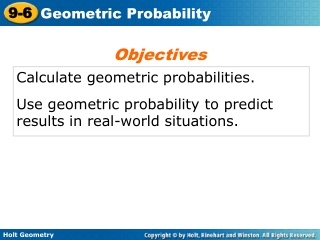DownloadDownload PresentationCalculate geometric probabilities.

# Calculate geometric probabilities.

Télécharger la présentation## Calculate geometric probabilities.

- - - - - - - - - - - - - - - - - - - - - - - - - - - E N D - - - - - - - - - - - - - - - - - - - - - - - - - - -
##### Presentation Transcript

1. Objectives Calculate geometric probabilities. Use geometric probability to predict results in real-world situations.

2. If every outcome in the sample space is equally likely, the theoretical probability of an event is Remember that in probability, the set of all possible outcomes of an experiment is called the sample space. Any set of outcomes is called an event.

3. Remember! If an event has a probability p of occurring, the probability of the event not occurring is 1 – p.

4. The point is on RS. Example 1A: Using Length to Find Geometric Probability A point is chosen randomly on PS. Find the probability of each event.

5. The point is not on QR. Subtract from 1 to find the probability that the point is not on QR. Example 1B: Using Length to Find Geometric Probability

6. P(PQ or QR) = P(PQ) + P(QR) Example 1C: Using Length to Find Geometric Probability The point is on PQ or QR.

7. Use the figure below to find the probability that the point is on BD. Check It Out! Example 1

8. Example 2A: Transportation Application A pedestrian signal at a crosswalk has the following cycle: “WALK” for 45 seconds and “DON’T WALK” for 70 seconds. What is the probability the signal will show “WALK” when you arrive? To find the probability, draw a segment to represent the number of seconds that each signal is on. The signal is “WALK” for 45 out of every 115 seconds.

9. Example 3A: Using Angle Measures to Find Geometric Probability Use the spinner to find the probability of each event. the pointer landing on yellow The angle measure in the yellow region is 140°.

10. Example 3B: Using Angle Measures to Find Geometric Probability Use the spinner to find the probability of each event. the pointer landing on blue or red The angle measure in the blue region is 52°. The angle measure in the red region is 60°.

11. Example 3C: Using Angle Measures to Find Geometric Probability Use the spinner to find the probability of each event. the pointer not landing on green The angle measure in the green region is 108°. Subtract this angle measure from 360°.

12. Example 4: Using Area to find Geometric Probability Find the probability that a point chosen randomly inside the rectangle is in each shape. Round to the nearest hundredth.

13. ≈ 0.18. The probability is P = 254.5 1400 Example 4A: Using Area to find Geometric Probability the circle The area of the circle is A = r2 = (9)2 =81 ≈ 254.5 ft2. The area of the rectangle is A = bh = 50(28)=1400 ft2.

14. The area of the trapezoid is The probability is Example 4B: Using Area to find Geometric Probability the trapezoid The area of the rectangle is A = bh = 50(28)=1400 ft2.

15. The probability is Example 4C: Using Area to find Geometric Probability one of the two squares The area of the two squares is A = 2s2 = 2(10)2 =200 ft2. The area of the rectangle is A = bh = 50(28)=1400 ft2.